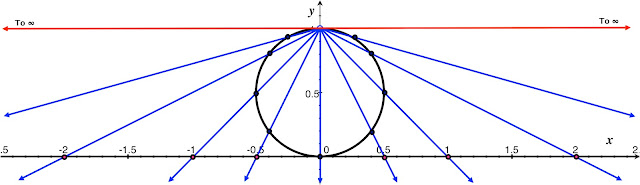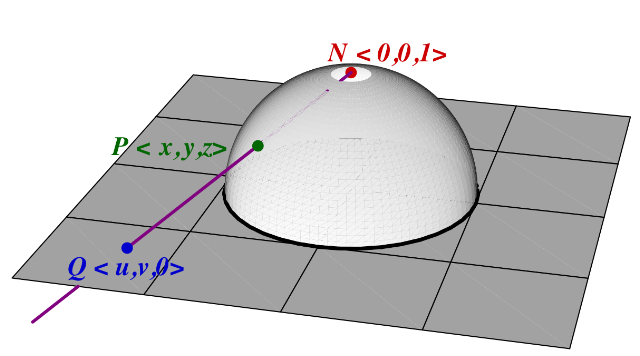## Tuesday, September 1, 2015

### Clifford Tori

As part of the ongoing celebration that is the relaunch, here, we finally give the long-awaited in-depth look at the site's namesake hinted at in the opening post. Perhaps surprisingly in a post that ostensibly is about tori, we begin our story with a different well-known (and hopefully loved) family of spaces: the spheres. The $1$- and $2$-dimensional spheres hardly need introduction, being undoubtely the first curved geometrical objects that one studies, the ordinary circle and sphere (which for mathematicians, only refers to the boundary surface, not the interior, of a ball, so is $2$-dimensional). These shapes of course appear all the time in nature, since they satisfy many optimality properties. Much, of course, has been written about their "perfect form."

Higher-dimensional spheres are easy to define: the set of all points a given distance from a given point. Where, of course, "all points" start out as being in some higher dimensional space, say, n. High-dimensional spheres have interesting applications, such as in statistical mechanics, where the dimension of the state space in question is on the order of $10^{24}$ (every particle gets its own set of 3 dimensions! Again, this is why state space is awesome). Spheres occur in this context because the total energy of the system remains constant, so the sum of the squares of all their momenta has to be constant—namely, the momenta are all a certain "distance" from the origin. As crazy as it may sound, it is, at its root, a description (using Hamiltonian mechanics) of many more than the two particles that we spent a whole 5-part series talking about! But of course, it'd take us a bit far afield to explore this (at least in this post; we should eventually feature some statistical-mechanical calculations here, because it is illustrative of how we can deal with overwhelmingly large-dimensional systems and, rather amazingly, be able to extract useful information from it!).

So let's get back to nearly familiar territory: $n = 3$, the $3$-sphere $S^3$ (the boundary of a ball in 4-dimensional space $\mathbb{R}^4$). Being $3$-dimensional, one would think we can visualize it or experience it viscerally somehow. As noted by Bill Thurston, the key to visualization of $3$-manifolds is to imagine living inside a universe that is shaped like one (this is also elaborated upon, working up from $2$-dimensional examples, by Jeffrey Weeks, with interactive demos). This isn't so straightforward to visualize (literally), because it is a curved 3-dimensional space. There are a number of ways of doing this; they give the essence of $S^3$ by understanding it in terms of some more familiar objects from lower dimensions, such as slicing by hyperplanes, and of course, by tori.

#### The Clifford Tori as a Foliation of $S^3$

So where do tori (nested or not) come in? Let's get back to the defining formula of $S^3$,
$x_1^2 + x_2^2 + x_3^2 + x_4^2 = 1.$ If we consider groups of $2$ terms, $x_1^2 + x_2^2 = A^2$, and $x_3^2 + x_4^2 = B^2$, we have, $A^2 + B^2 = 1$. But for each fixed $A$ and $B$ satisfying that relation, we get two circles: one for the coordinates $(x_1,x_2)$ and another for the coordiantes $(x_3,x_4)$. We can use this information to help parametrize $S^3$. Let's first ask: what are some familiar things satisfying $A^2+B^2 = 1$? If we let $A = \cos \varphi$ and $B = \sin \varphi$, then $A$ and $B$ will always satisfy this relation for any $\varphi \in \mathbb{R}$: so $\varphi$ is one possible parameter of this system, and the full possible range of $A^2$ and $B^2$ is assumed by letting $\varphi$ vary from $0$ to $\frac \pi 2$. This gives us: $x_1^2 + x_2^2 =\cos^2 \varphi$ and $x_3^2 + x_4^2 = \sin^2 \varphi.$
In other words, as $\varphi$ varies, the two sets of coordinates represent one circle that grows in radius, and another that shrinks, in such a way that the sum of the squares of the two radii are always $1$. This realizes the $3$-sphere as a collection of sets of the form $S^1(A) \times S^1(B) = S^1(\cos\varphi) \times S^1(\sin\varphi)$, the Cartesian product of circles of radius $A$ and $B$. But what is the Cartesian product of two circles? A torus. These tori fill up all of $S^3$ (except for two degenerate cases: two circles, corresponding to the Cartesian product of a unit circle and a single point—a circle of radius $0$). The technical term for this is that they foliate $S^3$ (from the Latin folium for "leaf"). They are called Clifford tori. Hard as it may be to believe, their intrinsic geometry, as inherited from $\mathbb{R}^4$ is flat (although this is not true of their extrinsic geometry). It means that if we have a sheet of paper, we could lay it flat on a Clifford torus in $\mathbb{R}^4$ (you can't do that in $\mathbb{R}^3$ with your garden-variety donut-shaped torus). However, a full study of the geometry of these tori will have to wait for another time. Here, we will be content to visualize them (which, unfortunately, will not preserve that flat geometry we are claiming they have). For the visualization, we use another tool, the stereographic projection. Before we get to that, we finally note that, for each given $\varphi$, each of the circles $S^1(\cos \varphi)$ and $S^1(\sin\varphi)$ can be further parametrized with other angles; we take
$(x_1,x_2) = (\cos \alpha \cos \varphi, \sin \alpha \cos \varphi)$ and $(x_3,x_4) = (\cos \beta \sin \varphi, \sin \beta \sin \varphi),$
where $0\leq \alpha,\beta \leq 2\pi$. So this means we have parametrized $S^3$ by 3 variables, $(\varphi,\alpha,\beta)$ varying over $[0,\pi/2]\times [0,2\pi]\times [0,2\pi]$.

#### The Stereographic Projection

There's a way to project (almost) the whole $3$-sphere into ordinary $3$-space $\mathbb{R}^3$. To understand how we can project the $3$-sphere (minus a point) into $3$-space, let's look at the analogous problem for the $2$-sphere, first. It so happens that the stereographic projection is extremely useful in that case as well, and is the source for many arguments involving "the point at infinity" in complex analysis. The way it works is to imagine screwing in a light bulb at the top of a sphere resting on a plane, and given a point on a sphere, its shadow cast on the plane by this light is the corresponding plane (shown in the figure below for a line: the dots connected by the blue rays correspond).Some corresponding points for the stereographic projection of a circle (here of radius $\frac 1 2$) to a line.
Notice that the closer it gets to the top, the farther out the rays go (thus, the farther out the corresponding point). And the top point falls on a horizontal line, which will never intersect the corresponding plane of projection: it is said to be sent to the "point at infinity". But the thing is, that extra point at infinity is, at least for the plane as we've defined it, truly extra, so the stereographic projection really simply maps the sphere minus one single point to the plane. In formulas, for a sphere of radius $\frac{1}{2}$ centered at $(0,0,\frac{1}{2})$, this is
$\left(\frac{x}{1-z},\frac{y}{1-z}\right).$
Of course, if we want to map the unit (radius 1 and origin-centered) sphere, we have to do a little finessing with an extra transformation, namely, $(x',y',z') = (2x, 2y, 2z-1)$. It so happens that we get the exact same formula back, just a different domain:
$\left(\frac{x}{1-z},\frac{y}{1-z}\right) = \left(\frac{\frac{1}{2}x'}{1-\frac{1}{2}z'-\frac{1}{2}},\frac{\frac{1}{2}y'}{1-\frac{1}{2}z'-\frac{1}{2}}\right) =\left(\frac{x'}{1-z'},\frac{y'}{1-z'}\right).$
For this reason, many people start off with this formula for the unit sphere instead. The picture associated to this actually is nice: it projects rays of light from the top, through the sphere, and onto a plane that slices the sphere exactly in half at its equator. The consequence is that the light hits the corresponding point in the plane first before reaching a point in the lower hemisphere (but, mathematically, we keep the mapping as always from the sphere to the plane, regardless which the light beam would hit first). It should also be noted that the inverse mapping, of course, will be different (we won't be needing the inverse mapping here, but it is also useful in other contexts, and can be derived using elementary, if tedious, means, via messy algebra).Stereographic projection of the unit, origin-centered sphere to the plane containing its equator. The projection associates the point $P$ to the point $Q$.

So we generalize this formula: for the unit 3-sphere, given as $x_1^2 + x_2^2 + x_3^2 + x_4^2 = 1$, we have a stereographic projection $P$ of all of its points except the point $(0,0,0,1)$, to $\mathbb{R}^3$, as follows:
$P \begin{pmatrix} x_1 \\ x_2 \\ x_3 \\ x_4\end{pmatrix} =\frac 1 {1-x_4}\begin{pmatrix} x_1\\ x_2 \\ x_3\end{pmatrix}.$
In order to visualize our Clifford Tori, then, we recall the parametrization derived above:
$\begin{pmatrix} x_1 \\ x_2 \\ x_3 \\ x_4\end{pmatrix} = F\begin{pmatrix}\varphi \\ \alpha \\ \beta\end{pmatrix} = \begin{pmatrix} \cos \alpha \cos \varphi \\ \sin\alpha\cos\varphi \\ \cos\beta\sin\varphi \\ \sin\beta\sin\varphi \end{pmatrix}.$
The two circles that we want are when $\alpha$ and $\beta$ vary, so if we select a few values of $\varphi$ and draw the surface by the parametrization with the remaining variables, we will get different tori for each value of $\varphi$. But, of course, this still gives the torus as being in $\mathbb R^4$. So we compose this with the stereographic projection: for fixed $\varphi$, and letting $\alpha, \beta$ vary, we consider parametrizations
$\Phi \begin{pmatrix} \varphi \\ \alpha \\ \beta \end{pmatrix} = P \circ F\begin{pmatrix} \varphi \\ \alpha \\ \beta \end{pmatrix} = \frac{1}{\sin\beta\sin\varphi} \begin{pmatrix} \cos \alpha \cos \varphi \\ \sin\alpha\cos\varphi \\ \cos\beta\sin\varphi \end{pmatrix}.$

These are the tori given in the opening post (the tori are deliberately shown incomplete, with $\beta$ not going full circle, so that you can see how they change with different $\varphi$ and that they are nested. Note that this transformation does not preserve sizes, so even though we said that "one circle gets bigger as the other gets smaller", this is not what happens in the projection. Think of a sphere with circles of latitude; they grow from one pole to the equator and shrink back down from the equator to the other pole, but their stereographic projections to the plane just keep growing. The other view of this, and the site's logo, is simply a cutaway view of the stereographic projections by one vertical plane. As a note of thanks, bridging years, one of the sources of inspiration for learning about Clifford tori and their visualizations has come from Ivars Peterson's The Mathematical Tourist. (He maintains a blog as well).

We should finally note that this is not the same parametrization as our usual torus of revolution. Parametrizations are not unique. The Clifford tori have many interesting, topologically important properties that will certainly be fodder for future posts.

#### 1 comment:

1.Hi Chris, thanks for the interesting overview. So we can write the line element on S^3 as ds^2 = (1-r^2/l^2) d\tau^2 + (1-r^2/l^2)^{-1} dr^2 + r^2 d\phi^2 - this is basically the metric on the static patch of de Sitter space analytically continued to Euclidean signature. The surfaces of constant r in these coordinates are tori where the circles are parameterized by \phi and \tau (which is also a periodic coordinate). Are these tori Clifford tori??